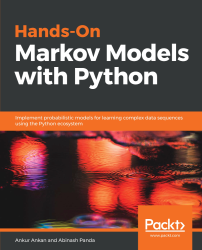•#### Hands-On Markov Models with Python#### Overview of this book

Hidden Markov Model (HMM) is a statistical model based on the Markov chain concept. Hands-On Markov Models with Python helps you get to grips with HMMs and different inference algorithms by working on real-world problems. The hands-on examples explored in the book help you simplify the process flow in machine learning by using Markov model concepts, thereby making it accessible to everyone. Once you’ve covered the basic concepts of Markov chains, you’ll get insights into Markov processes, models, and types with the help of practical examples. After grasping these fundamentals, you’ll move on to learning about the different algorithms used in inferences and applying them in state and parameter inference. In addition to this, you’ll explore the Bayesian approach of inference and learn how to apply it in HMMs. In further chapters, you’ll discover how to use HMMs in time series analysis and natural language processing (NLP) using Python. You’ll also learn to apply HMM to image processing using 2D-HMM to segment images. Finally, you’ll understand how to apply HMM for reinforcement learning (RL) with the help of Q-Learning, and use this technique for single-stock and multi-stock algorithmic trading. By the end of this book, you will have grasped how to build your own Markov and hidden Markov models on complex datasets in order to apply them to projects.
PrefaceFree Chapter
Introduction to the Markov ProcessHidden Markov ModelsState Inference - Predicting the StatesParameter Learning Using Maximum LikelihoodParameter Inference Using the Bayesian ApproachTime Series PredictingNatural Language Processing2D HMM for Image ProcessingMarkov Decision ProcessOther Books You May Enjoy# 2D HMM for Image Processing

In this chapter, we will introduce the application of HMM in the case of image segmentation. For image segmentation, we usually split up the given image into multiple blocks of equal size and then perform an estimation for each of these blocks. However, these algorithms usually ignore the contextual information from the neighboring blocks. To deal with that issue, 2D HMMs were introduced, which consider feature vectors to be dependent through an underlying 2D Markovian mesh. In this chapter, we will discuss how these 2D HMMs work and will derive parameter estimation algorithms for them. In this chapter, we will discuss the following topics:

• Pseudo 2D HMMs
• Introduction to 2D HMMs
• Parameter learning in 2D HMMs
• Applications